Next: References

# Critical remarks on the antimonotonicity theorem

Kai T. Hansen

We will here review and criticise some arguments given in

ref. [KKY] by Kan, Koçak, and Yorke. The main result in [KKY] is the antimonotonicity theorem stating the following:

In any neighborhood of a nondegenerate, homoclinic-tangency parameter value of a one-parameter family of dissipative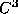diffeomorphisms of the plane, there must be both infinitely many orbit-creation and infinitely many orbit-annihilation parameter values.

We agree with [KKY] that there will exist some one-parameter families with both orbit-creations and orbit-annihilations, but we do not agree that all one-parameter families must have this property. We believe that there will exist cones in a high dimensional parameter space which include one-parameter families where orbits are only created as the parameter increases. We will analyse here the arguments in [KKY], and conclude that the arguments used here are not sufficient to claim that all one-parameter families are antimonotone.

The authors of [KKY] use a relatively simple example to illustrate their arguments. The proof for the general case follows the same type of arguments as the specific example, so the argument that we find to fail in the specific case should also invalidate the proof of the general case. We will therefore discuss only the specific example given by Kan et al.

The model given in [KKY] is the map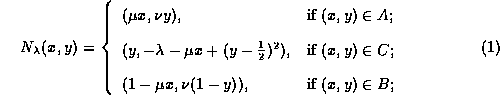The regions A, C, and B are given by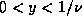,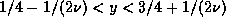, and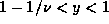respectively. We assume here that the border for region C should be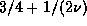rather than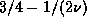as written in ref. [KKY]. This modification makes the map symmetric, but is otherwise unimportant for the arguments to follow. The number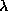is the parameter of the map whileand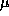are fixed numbers fulfilling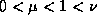and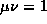. The figures shown here were evaluated with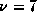.

Regardless of the value ofthis map will have one complete Cantor set of non-wandering orbits denoted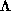. In addition there exist families of non-wandering orbits which are created or destroyed as the parameteris varied. The question raised in [KKY] is whether non-wandering orbits will be both created and destroyed asincreases, especially for small negative values of. The authors construct an example, discussed below, which is used to argue that there will be destroyed orbits asincreases. We find this argument not valid.

Figure 1 (a) shows the three regions A, C, and B. Figure 1 (b) shows the mapping of these regions. A part of the unstable and stable manifolds of the fixed point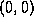are drawn in figure 2. The setis the closure of the crossings between the parts of the stable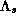and unstable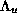manifolds ofwhich never leave the regions A and B. These manifolds are horizontal and vertical lines, not changing with changing parameter, and they can be labelled using a binary symbolic dynamics, given by the order in which the trajectory visits the regions A and B.

The image of one vertical line ofthrough the region C is a parabola with a minimum point at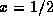. This parabola is in [KKY] denoted a primary unstable line segment and is parametrised as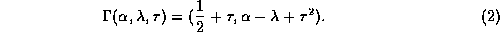Hereis a negative number corresponding to one point in the Cantor set of unstable manifolds, that is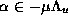. We remark here thatis not a function of. Ifdepends onthen this parabola is a part of the unstable manifold only for a specific parameter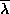and not for all parameters close to this, since close to any point in a Cantor set there will be an open gap.

Kan et al. choose to look at the case where the center part of the primary unstable parabola is mapped n times in region A and then enters the region C where the image of the parabola is a new narrow parabola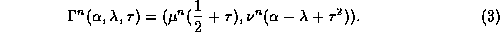If the minimum point of this parabola is larger than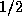then the next image of this fold is in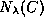and has one minimum point close to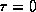. If the minimum point of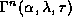is less thanand not too close tothen the image of the parabola has one maximum point close toand two minimum points.

The image of the narrow parabola is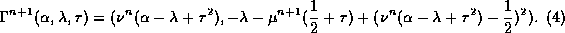The partial derivative of the y coordinate with respect tois then found to be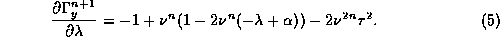We find that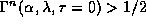if and only if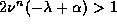and therefore have that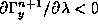. This implies that asincreases the minimum point decreases and new homoclinic orbits may be created. The minimum point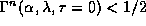if and only if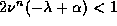. Since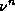is a large number we will have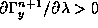unless the minimum point is very close tooris very different from zero. We then find that the maximum point increases withand can create new homoclinic orbits. The primary parabola with a minimum point goes downward with an increasingand also gives the possibility of creating new orbits without destroying any other orbits. The conclusion here is that all the manifolds discussed here only create and do not destroy orbits asincreases. This is illustrated in figure 2 where the manifolds move in this way.

This conclusion is the opposite of the conclusion in ref. [KKY], because the authors there do not consider parabolas with a fixed value ofbut letbe a function of. With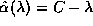where C depends on,, and n, they find that one obtains a curve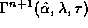with a maximum point that decreases with increasing. As we increasetwo crossing points betweenand a horizontal line belonging to the stable manifoldswill disappear in a point where the curves are tangents. The authors use density arguments to show that there will exist asuch that this tangency point is a homoclinic tangency between a stable and unstable manifold. We agree that if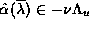then the tangency is a homoclinic tangency, but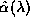for any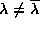is not guaranteed to be in the unstable manifold and especially will it not be the same fold as specified by e.g. a symbolic alphabet since the folds are fixed on the x-axis under changes of. How the crossings between the curve given byand a horizontal stable manifold change, tells us nothing about which orbits that are created in the non-wandering set. The authors claim that this proves that orbits are destroyed is therefore not valid. The homoclinic tangencies are for this example in fact contact-creating and not contact breaking as claimed in ref. [KKY].

We conclude that the Antimonotonicity theorem is not proven.

We have not succeeded in disproving the theorem, but believe that it is wrong as stated. In general one can presumably associate a parameter with the height of every primary unstable parabola, thus having a parameter space of an infinite, uncountable dimension. This parameter space set could be well approximated with a finite number of parameters if the manifolds form a not too dense Cantor set. We have done this [Han,HC] for the Hénon map where we have used symbolic dynamics to construct an approximate parameter space (assuming the existence of a symbolic dynamics for the maps of Hénon type). Bifurcation planes can be analytically found in this parameter space and there exist a large cone from every parameter point in which all one-dimensional parameter paths are monotone. These results are confirmed by numerics for the Hénon map. One observes non-monotonicity if one chooses a parameter path which is not inside a proper cone in the parameter space. Most one-dimensional parameter paths in a high dimensional parameter space will not be in a proper cone and one can therefore in numerical experiments expect to see both bifurcations and inverse bifurcations.Next: References

Kai Hansen
Tue Nov 26 14:45:50 MET 1996